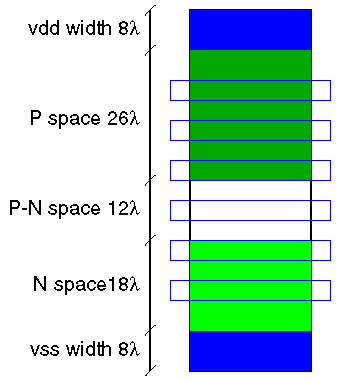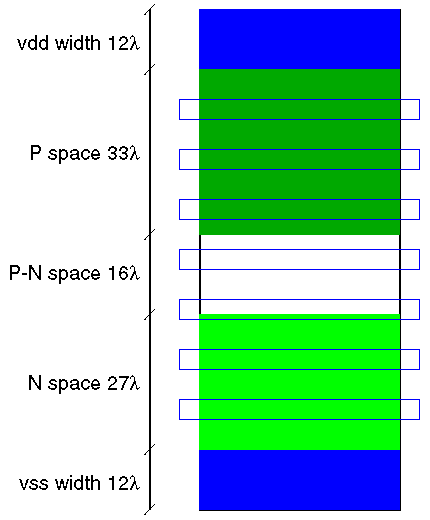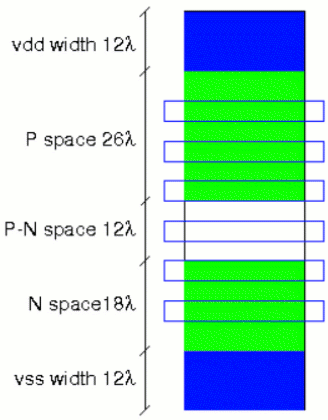The parameter ps is the percentage of metal-1 used for the standard cell power supplies and depends on the layout style used.

The drawing of the vsclib standard cell template above shows a cell height of 72 lambda with each supply 8 lambda wide. The amount of metal‑1 used for the power supplies is then

 ps = (8+8)⁄72 = 22.2%

The Alliance sxlib standard cell template at the top right shows each supply at 12 lambda wide and a total cell height of 100 lambda. The metal‑1 power supply percentage is then

 ps = (12+12)⁄100 = 24%

The wsclib standard cell library on the right is 80 lambda tall with 12 lambda wide Vdd and Vss power rails. The metal‑1 percentage used for power supplies is then

 ps = (12+12)⁄80 = 30%